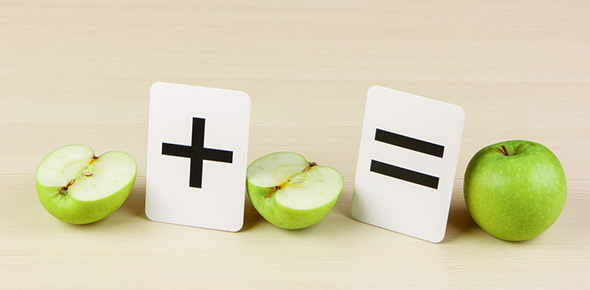# Fractions/ Decimals/ Percentages/ Ratios Pre Test

24 Questions | Attempts: 714
ShareSettings• 1.
Order these decimal from smallest to largest 0.25, 0.75, 1.5, 0.20, 0.5, 0.6
• 2.
Which is the equivalent fraction for 1/2?
• A.

4/9

• B.

2/4

• C.

3/9

• D.

5/8

• 3.
Which is the equivalent fraction for 3/5?
• A.

1/5

• B.

3/10

• C.

4/9

• D.

6/10

• 4.
How would you write this fraction in the simplest form 3/9?
• A.

1/3

• B.

3/6

• C.

1/9

• D.

3/9

• 5.
How would you write this fraction in the simplest form 12/24?
• A.

6/12

• B.

1/2

• C.

1/3

• D.

3/12

• 6.
Put these fractions in order from smallest to largest1/2, 1/5, 3/4, 1/4, 5/5
• 7.
Write this fraction as a decimal 1/2
• 8.
Write this fraction as a decimal 3/4
• 9.
Write this fraction as a decimal 7/10
• 10.
Write this fraction as a decimal 1/5
• 11.
Write this fraction as a decimal 1/4
• 12.
Write this fraction as a decimal 45/100
• 13.
Write this fraction as a decimal 1/25
• 14.
Write this fraction as a percentage 1/2
• 15.
Write this fraction as a percentage 3/4
• 16.
Write this fraction as a percentage 1/4
• 17.
Write this fraction as a percentage 1/5
• 18.
Write this fraction as a percentage 9/10
• 19.
Write this decimal as a percentage 0.30
• 20.
Write this decimal as a percentage 0.90
• 21.
Write this decimal as a percentage 0.75
• 22.
What do you know about fractions?
• 23.
What do you know about decimals?
• 24.
What do you know about percentages?

## Related TopicsBack to top
×

Wait!
Here's an interesting quiz for you.